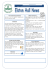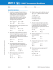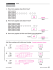Absolute Value

Transcription

Absolute Value
Name ________________________________________ Date __________________ Class __________________
LESSON
1-3
Absolute Value
Practice and Problem Solving: D
Graph each number on the number line. The first one is done for you.
1. −5
3. −7
2. 4
4. 5
Use the number line to find each absolute value. The first one is
done for you.
4
5. |4| _________________
6. |−5| _________________
7. |7| _________________
8. |5| _________________
9. |−4| _________________
10. |6| _________________
Complete.
11. The absolute values of −5 and 5 are the _________________.
12. The integers −5 and 5 are called _________________.
Use the table for exercises 13–22.
Temperatures at a Ski Resort
Monday
Tuesday Wednesday Thursday
5°F
2°F
below zero below zero
0°F
Friday
2°F
3°F
above zero below zero
Write a negative integer to show the amount each temperature is
below zero. The first one is done for you.
−5
13. 5°F below zero ____
14. 2°F below zero ____
15. 3°F below zero ____
16. Can 0°F be written as a negative integer? ____
Find the absolute value of each temperature below zero. The first one
is done for you.
5
17. |−5| = ___
18. |−2| = ____
19. |−3| = ____
Complete.
20. On which day was the temperature the coldest? _________________
21. On which day was the temperature the warmest? _________________
22. When a number is negative, its opposite is also its
_____________________________________.
Original content Copyright © by Houghton Mifflin Harcourt. Additions and changes to the original content are the responsibility of the instructor.
15
5. 4
LESSON 1-3
6. 5
Practice and Problem Solving: A/B
7. 7
1−4.
8. 5
9. 4
5. 6
10. 6
6. 3
11. same
7. 8
12. opposites
8. 6
13. −5
9. 3
14. −2
10. 5
15. −3
11. The absolute values of 6 and −6 are
the same.
16. no
12. opposites
17. 5
13. −20
18. 2
19. 3
14. −6
20. Monday
15. −8
21. Thursday
16. 20
22. absolute value
17. 6
Reteach
18. 15
19. Monday; the greatest negative number
shows the greatest amount spent.
1. c
20. |3 + 10| = |13| = 13; |3| = 3 and |10| =
10; 3 + 10 = 13; 13 = 13
3. b
21. two; possible answer: −4 and 4
5. c
2. a or d
4. a
Practice and Problem Solving: C
6. 3
1. 2,300
7. 5
2. Elan; 2,910
8. 7
3. Pietro; 2,080
9. 6
4. Bill, Jorge
10. 0
5. Bill, Jorge
11. 2
6. Elan
12. 10
7. +186
13.
8. 324
3
4
14. 0.8
9. Their absolute values are the same.
15. Sample answer: The absolute value of a
number is the number’s distance from 0
on the number line. Since the distance is
positive or 0, absolute value is always
positive or 0. For example |−5| = 5 and
|5| = 5.
10. −125 and 125; Negative deviations would
decrease by 100; positive differences
would increase by 100.
Practice and Problem Solving: D
1−4.
Original content Copyright © by Houghton Mifflin Harcourt. Additions and changes to the original content are the responsibility of the instructor.
349

Birmingham News Article - Monday Morning Quarterback Club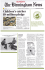Music Matching Game Music Matching Game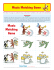Lesson 1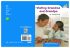Children`s & Teen Author Speed Dating Event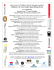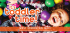Lesson 8:In the Rain Forest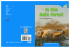Unit Four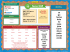Newsletter 18th May 2015 - Elston Hall Primary School